# 1st PUC Chemistry Question Bank Chapter 9 Hydrogen

Students can Download Chemistry Chapter 9 Hydrogen Questions and Answers, Notes Pdf, 1st PUC Chemistry Question Bank with Answers helps you to revise the complete Karnataka State Board Syllabus and to clear all their doubts, score well in final exams

## Karnataka 1st PUC Chemistry Question Bank Chapter 9 Hydrogen

Introduction

Question 1.
What is the present position of hydrogen the periodic table?
Hydrogen is the first element in the periodic table and placed along with alkali metals.

Question 2.
The position of hydrogen in the periodic table is still uncertain. Give reasons.
Hydrogen has resemblance to alkali metals, and can lose one electron to form unipositive ions, as well as with halogens, and can gain one electron to form uninegative ion. Properties of hydrogen do not completely match any one of the above-mentioned groups. That is why position of hydrogen is still uncertain.

Question 3.
Which is the principal element in the solar atmosphere?
DihydrogenDihydrogen

Question 1.
Name the most abundant element in the universe.
Dihydrogen

Question 2.
What is the percentage of dihydrogen present in the universe?
70%.

Question 3.
Name the planets made up of mostly hydrogen.
Jupiter and Saturn.

Question 4.
The percentage of dihydrogen is less in Earth’s atmosphere. Why?
Due to the light weight of dihydrogen.

Question 5.
Name three isotopes of hydrogen.
Protium (11H), Deuterium (21H or D) and tritium (31H or 31T)

Question 6.
Name the radioactive isotope of hydrogen.
Tritium (1H3)
Note:

• Isotopes of hydrogen have same chemical properties due to their same electronic configuration.
• The rates of reactions of isotopes of hydrogen are different due to their different enthalpy of bond dissociation.
• Physical properties of isotopes of hydrogen are different due to their large difference in their masses.

Preparation of Dihydrogen (H2)

Question 1.
Give the laboratory methods of preparation of dihydrogen.
(a) Hydrogen is prepared in the laboratory by the action of Zn with dilute hydrochloric acid.
Zn + 2HCl → ZnCl2 + H2
(b) Hydrogen can also be prepared by the reaction of zinc with aqueous NaOH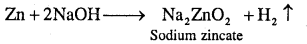Question 2.
Give the commercial method of production of dihydrogen.
(a) Commercially, hydrogen gas is obtained by the electrolysis of acidified water using platinum electrode.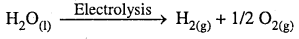(b) High purity dihydrogen gas (> 99.95%) is obtained by electrolysing warm aqueous barium hydroxide solution between nickel electrodes.
(c) Dihydrogen gas is also obtained as by product during the manufacture of sodium hydroxide and chlorine by the electrolysis (Nelson’s cell) of brine solution (saturated aqueous solution of NaCl is called brine solution).

The cell reactions during electrolysis are
At anode: 2Cl (aq) → Cl2(g) + 2e
At cathode: 2H2O(l) + 2e → H2(g) + 2OH(aq)
The overall reaction is
2Na+ (aq) + 2Cl (aq) + 2H2O(1) → Cl2 (g) + H2 (g) + 2Na+(aq) + 2OH (aq)Question 3.
How do you prepare dihydrogen from coke and hydrocarbons?
Dihydrogen is obtained by the action of steam with hydrocarbons or coke at high temperatures (1270 °K).
For example,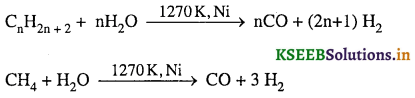Question 4.
What is water gas? What is its use?
The mixture of CO and H2 is called water gas. It is used for the synthesis of methanol and a number of hydrocarbons.

Question 5.
Why is water gas also called synthesis gas or syngas?
Because water gas is used in the synthesis of methyl alcohol and a number of hydrocarbons.

Question 6.
What is coalgasification?
The process of producing syngas from coal gas is called coalgasification.
C + H2O → CO + H2

Question 7.
What is water-gas shift reaction?
The production of dihydrogen can be increased by the reaction CO of syngas with steam in the presence of iron chromate catalyst is called water gas shift reaction.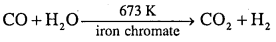Properties of Dihydrogen

Question 1.
Dihydrogen is relatively inert at room temperature. Give reason.
Due to high H – H bond enthalpy.

Question 2.
How does dihydrogen reacts with halogens? Give equation.
Dihydrogen combines with halogens (X) to give hydrogen halides. The reactivity of halogens towards dihydrogen decreases in the order F2 > Cl2 > Br2 > I2.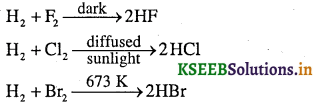Question 3.
Write the reactions of dihydrogen with dioxygen and dinitrogen.
Hydrogen reacts with dioxygen to form water. The reaction is highly exothermic.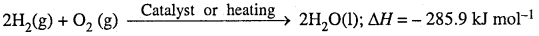Hydrogen reacts with dinitrogen in the presence of iron catalyst at 673 K under a pressure of 200 atm to form ammonia. This is the method of manufacture of ammonia by Haber process.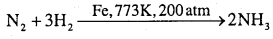Question 4.
Give the reaction of dihydrogen with metals.
Dihydrogen combines with many metals at high temperatures to yield the corresponding metal hydrides.
H2 + M → 2MH where M is alkali metal.

Question 5.
Give the reactions of dihydrogen with metal ions and metal oxides.
Dihydrogen gas reduces some metal ions in aqueous solution and oxides of metals into corresponding metals.
H2 (g) + Pd2+ (aq) → Pd(s) + 2H+ (aq)
yH2(g) + MxOy(s) → xM(s) + yH2O(l)

Question 6.
Explain the reactions of dihydrogen with organic compounds with examples.
Dihydrogen reacts with many organic compounds in the presence of catalyst to give useful hydrogenated products.
For example:
a. Hydrogenation of vegetable oils using Ni catalyst gives edible fats (dalda or vanaspathi ghee).b. Hydroformylation of alkenes (olefins) gives aldehydes which further reduction gives alcoholsQuestion 7.
Comment on the reactions of dihydrogen with (i) Chlorine, (ii) Sodium, and (iii) Copper (II) Oxide
(i) Dihydrogen reduces chlorine into chloride (Cl) and itself oxidised to H+ ion by chlorine to form hydrogen chloride. ‘
H2 + Cl2 → 2HCl (covalent molecule)

(ii) Dihydrogen is reduced by sodium to form sodium hydride.
H2 + 2Na → 2Na+H (ionic compound)

(iii) Dihydrogen reduces copper (II) oxide to copper (0) and itself oxidised to water.
CuO + H2 → CU + H2OQuestion 8.
Give any four uses of dihydrogen.

1. It is used in the production of ammonia by Haber’s process.
2. Hydrogen is commonly used in power stations, as a coolant for generators.
3. It is used in the manufacture of vanaspathi by hydrogenation of vegetable oil.
4. The largest application of H2 is for the processing of fossil fuels.
5. Hydrogen is used as a shielding gas in welding methods such as atomic hydrogen welding.
6. It is used as a rocket fuel in space research.
7. Hydrogen is used in the manufacture of methanol.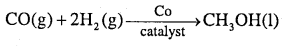Question 9.
What are hydrides? Give the classification of hydrides.
Binary compounds of hydrogen are known as hydrides. If ‘E’ is the symbol of an element then hydride can be expressed as EHx (example, MgH2) or EmHn (example, B2H6).
Hydrides are broadly classified into the following three types.

1. Ionic hydrides or saline hydrides
2. Covalent hydrides or molecular hydrides.
3. Metallic hydrides or nonstoichiometric or interstitial hydrides

Hydrides

Question 1.
What are ionic hydrides? Give examples.
These are stoichiometric compounds of dihydrogen formed with most of the s-block elements which are highly electropositive in character. Elements of group 1 and 2 except lighter metals Li, Be and Mg form ionic hydrides. Ionic hydrides are also known as saline hydrides or salt-like hydrides because their properties are identical to salts. In, ionic hydrides, hydrogen exists as H. For the hydrides of group 1, general formula is MH where M represents Na, K, Rb, Cs and Fr.
Examples, NaH, KH, RbH, etc.
For the hydrides of group 2, general formula is MH2 where M represents Ca, Sr and Ba. Examples, CaH2, SrH2, BaH2, etc.

Question 2.
Write a note on covalent hydrides or molecular hydrides. Give examples.
Elements of group 13 to 17 form covalent hydrides.
For example, BH3, AlH3, CH4, NH3, H2S, H2O or HCl.
Molecular hydrides are further classified into three types according to the relative numbers of electrons and bonds in their Lewis structure.

i. Electron-deficient hydrides
ii. Electron-precise hydrides
iii. Electron-rich hydrides

Electron-deficient hydrides: have less number of electrons than required for writing its conventional Lewis structure.
All elements of group 13 will form electron-deficient hydrides. For example, diborane (B2H6). They act as Lewis acids, i.e., electron pair acceptors.

Electron-precise hydrides: have the required number of electrons to write their conventional Lewis structures. All elements of group 14 form electron precise hydrides (example, CH4) which are tetrahedral in geometry.

Electron-rich hydrides: have excess electrons which are present as lone pairs. Elements of group 15-17 form such compounds (NH3 has one lone pair, H2O – two and HF -three lone pairs).

They behave as Lewis bases, i.e., electron pair donors. The presence of lone pairs on highly electronegative atoms like N, O and F in hydrides results in hydrogen bond formation between the molecules. This leads to the association of molecules.

Note: Due to higher electronegativity of N, O and F, the magnitude of hydrogen bonding in their hydrides will be quite appreciable. Hence, the boiling points of NH3, H2O and HF will be higher than the hydrides of their subsequent group members.Question 3.
What are metallic hydrides? Give examples.
These are the hydrides of transition elements (except elements of group 7, 8 and 9) and inner transitional elements. Hydrogen gas gets absorbed in empty spaces of d or f block elements to produce non-stoichiometric hydrides or interstitial hydrides which are known as metallic hydrides.
Examples, ZrH1.92, TaH0.76, PrH2.3, TiH1.7, LaH2.87, YbH2.55, TiH1.5-1.8, ZrH1.3-1.75, VH0.56, NiH0.6-0.7, PdH0.6-0.8

Question 4.
The property of adsorption of hydrogen gas on transition metal is used as catalyst and source of energy. Explain.
The property of adsorption of hydrogen gas on transition metals is widely used in catalytic reduction / hydrogenation reactions.for the preparation of large number of compounds. Some of the metals (example, Pd, Pt) can accommodate a very large volume of hydrogen and, therefore, can be used as its storage media. This property has high potential for hydrogen storage and as a source of energy.

Water

Question 1.
What is the approximate percentage of water in human body?
Human body – ≈ 65%.

Question 2.
Water has high boiling point, freezing point, high heat of vapourisation and heat of fusion compared to H2S and H2Se. Why?
Due to hydrogen bonding.

Question 3.
Write the structure of water molecule in the gas phase.
A molecule of water consists of two hydrogen atoms joined to an oxygen atom by covalent bonds.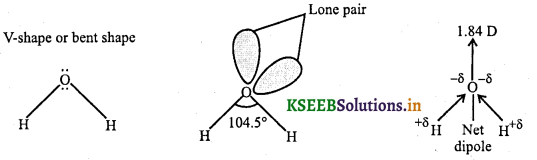Question 4.
Explain the amphoteric nature of water.
Water can act as both Bronsted acid as well as Bronsted base. This dual nature is called amphoteric nature. When HCl is dissolved in water, it reacts completely to form the hydronium ion, H3O+, and chloride ion.When, the ammonia gas is dissolved in water, some of the ammonia reacts with water to form the ammonium ion, NH+4, and hydroxide ion, OH.The self-ionization of water is another example of water being able to act both as an acid and as a base. One water molecule acts as an acid and the other acts as a base. The solution is neutral because equal quantities of H3O+ and OH are produced.
H2O + H2O → OH + H3O+

Question 5.
Explain redox reactions involving water with examples.
(a) Water reacts with highly electropositive metals like Na, K, etc. to liberate hydrogen gas.
2H2O(1) + 2Na(s) → 2NaOH(aq) + H2(g)

(b) During photosynthesis, water is oxidised to O2.
6CO2(g) + 12H2O(1) → C6H12O6(aq) + 6H2O(1) + 6O2(g)

(c) Highly electronegative flourine also oxidizes water to oxygen
2F2(g) + 2H2O(1) → 4HF(aq) + O2(g)

Question 6.
Complete the following reactions,
(a) P4O10 + 6H2O → ____
(b) SiCl4 + ____ → SiO2 + 4HCl
(c) N3- + 3H2O →______ + 3OH
(a) 4H3PO4
(b) 2H2O
(c) NH3Question 7.
Water has high hydrating tendency. Why?
Due to high dielectric constant.

Question 8.
Give an example for each
(a) Coordinated water
(b) Interstitial water
(c) Hydrogen bonded water.
(a) Coordinated water – [Cr(H2O)6]3+ 3Cl
(b) Interstitial water – BaCl2 .2H2O
(c) Hydrogen bonded water – [Cu(H2O)4]2+SO4.H2O

Question 9.
What is hard water?
Presence of calcium and magnesium salts in the form of bicarbonates chlorides and sulphates in water is called hard water.

Question 10.
What is soft water?
Water free from soluble salts of calcium and magnesium ions is called Soft water.

Question 11.
Give the difference between hard and soft water.

 Sl.NO. Hard water Soft water a. Presence of calcium and magnesium salts in the form of hydrogen carbonate, chloride and sulphate. Water free from soluble salts of calcium and magnesium ions. b. It gives less lather with soap. It gives more lather with soaps. c. Hard water forms scum/precipitate with soap. Soft water does not form scum/precipitate with soap.

Question 12.
Name the types of hard water.
There are two types of hard water.
a. Temporary hard water
b. Permanent hard waterQuestion 13.
Temporary hardness of water is due to what?
Due to the presence of magnesium and calcium hydrogen carbonate (bicarbonates).

Question 14.
Explain the process of softening of temporary hardness by heating.
Temporary hard water can be softned by heating. On heating Ca(HCO3)2 is converted into less soluble CaCO3 and therefore most of Ca2+ are precipitated as CaCO3. Mg(HCO3)2 is converted into Mg(OH)2 due to hydrolysis of MgCO3 formed. Mg(OH)2 being less soluble than MgCO3, most Mg2+ ions are precipitated as Mg(OH)2.
Mg (HCO3)2 → Mg (OH)2 + 2CO2
2Ca(HCO3)2 → 2CaCO3 + 2H2O + 2CO2
The insoluble precipitates can be removed by filtration.

Question 15.
Explain the process of softening of temporary hardness by Clark’s method.
Temporary hard water is converted into soft water by adding required amount of calcium hydroxide. In this method Mg2+ ions are precipitated as Mg(OH)2 and Ca2+ ions are precipitated as CaCO3.
Ca (HCO3)2 + Ca (OH)2 → 2CaCO3 + 2H2O
Mg (HCO3)2 + 2Ca (OH)2 → 2CaCO3 + Mg (OH)2 + 2H2O

Question 16.
Permanent hardness of water is due to what?
Due to the presence of soluble salts of magnesium and calcium in the form of chlorides and sulphates in water.

Question 17.
Explain the softening of permanent hardness of water by washing soda.
Washing soda reacts with soluble calcium and magnesium chlorides and sulphates in hard water to form insoluble carbonates.
MgCl2 + Na2CO3 → MgCO3 ↓ + 2NaCl ↓
MgSO4 + Na2CO3 → MgCO3 ↓ + Na2SO4
CaCl2 + Na2CO3 → CaCO3 ↓ + 2NaCl ↓
CaSO4 + Na2CO3 → CaCO3 ↓ + Na2SO4Question 18.
Explain softening of permanent hardness of water by Calgon’s process.
Calgon is a trade name of a complex salt, sodium hexametaphosphate (NaPO3)6. It is used for softening hard water. Calgon ionizes to give a complex anion:The addition of Calgon to hard water causes the calcium and magnesium ions of hard water to displace sodium ions from the anion of Calgon.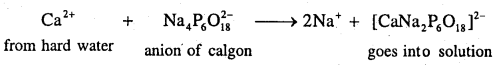This results in the removal of calcium and magnesium ions from hard water in the form of a complex with Calgon. The water is softened and sodium ions are released into water.

Question 19.
Explain the softening of hard water by ion exchange method.
Ion exchange version is made up of sodium aluminum silicate (NaAlSiO4) called zeolite or permutit. When this is added to hard water, ion exchange reactions takes place.
2NaZ + M2+ → MZ2 + 2Na+
Where M = Ca or Mg, Z = NaAlSiO4
Note: Zeolite can be regenerated for further use by treating with aqueous sodium chloride solution.
MZ2 + 2NaCl → 2NaZ + MCl2

Question 20.
Explain the process of softening of water by synthetic resin method.
Cation exchange resins contain large organic molecule with – SO3H group which are water insoluble. Ion exchange resin (RSO3H) is changed to RNa by treating it with NaCl. The resin exchanges Na+ ions with Ca2+ and Mg2+ ions present in hard water to make the water soft. Here R is resin anion.
2RNa(s) + M2+(aq) → R2M(s) + 2Na+(aq)
2RNa(s) + Mg2+(aq) → R2Mg(s) + 2Na+(aq)
The resin can be regenerated by adding aqueous NaCl solution. Pure de-ionized water free from all soluble mineral salts is obtained by passing water successively through a cation exchange (in the H+ form) and anion exchange (in the OH form) resins:
2RH(s) + Mg2+(aq) → MgR2(s) + 2H+(aq)
In this cation exchange process, H+ exchanges for Na+, Ca2+, Mg2+ and other cations present in water. This process results in proton release and thus makes the water acidic.
In the anion exchange process:
RNH2(S) + H2O(1) ⇌ RNH3+ ; OH(s)
RNH3+ . OH(s) + X(aq) ⇌ RNH+3 . X(s) + OH(aq)
OH exchanges for anions X = Cl, HCO3, SO2-4, etc. present in water. The liberated OH ions neutralise the H+ ions to form water.
H+(aq) + OH(aq) → H2O(1)
The exhausted cation and anion exchange resin beds are regenerated by treatment with dilute acid and alkali solutions respectively.Hydrogen Peroxide

Question 1.
Give the methods of preparation of hydrogen peroxide.
(i) Hydrogen peroxide is prepared by the action of sulphuric acid or phosphoric acid on hydrated barium peroxide BaO2 . 8H2O
BaO2.8H2O(s) + H2SO4(aq) → BaSO4(s) + H2O2(aq) + 8H2O(1)

(ii) Hydrolysis of peroxodisulphate (obtained by electrolytic oxidation of acidified sulphate solutions at high current density) gives hydrogen peroxide.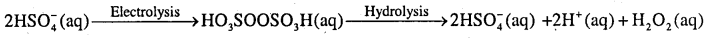Question 2.
Write a note on volume strength of hydrogen peroxide.
H2O2 is miscible in water in all proportions and forms a hydrate, H2O2.H2O (mp 221K). A 30% solution of H2O2 is marketed as 100 volume’ hydrogen peroxide or perhydrol. It means that one milliliter of 30% H2O2 solution will give 100 V of oxygen at STP. Commercially, H2O2 is marketed as 10 Volume, which means it contains 3% H2O2.

Question 3.
Calculate the strength of 10 volume solution of hydrogen peroxide.
10 volume solution of H2O2 means that 1 L of this H2O2 solution will give 10 L of oxygen at STP.On the basis of above equation 22.7 L of O2 is produced from 68 g H2O2 at STP
10 L of O2 at STP is produced from $$\frac{68 \times 10}{22 \cdot 7} \mathrm{g}$$ = 29 . 9g = 30g H2O2
Therefore, strength of H2O2 in 10 volume H2O2 solution = 30 g/L = 3% H2O2 solution.

Question 4.
Explain the structure of hydrogen peroxide.
Hydrogen peroxide is non-linear, non-planar molecule. It has a open book structure. The -O-O- linkage is called peroxy linkage. The structure is shown.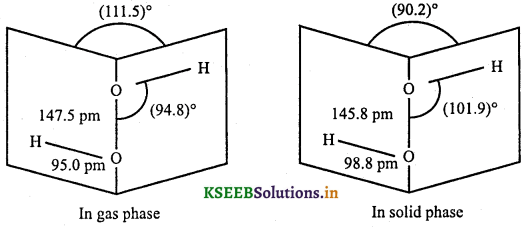Question 5.
Explain the oxidizing and reducing nature of H2O2 with examples.
1. Oxidising nature: It is a powerful oxidising agent. It acts as an oxidising agent in neutral, acidic or alkaline medium. Example,
PbS (s) + 4H2O2(aq) → PbSO4(s) + 4H2O(1)

2. Reducing nature: H2O2 has tendency to take up oxygen from strong oxidising agents and thus, acts as a reducing agent.
H2O2 + (O) → H2O + O2
Examples:
(i) Hydrogen peroxide reduces acidified permanganate ion to Mn2+.
2MnO4 + 6H+ + 5H2O2 → 2Mn2+ + 8H2O + 5O2

(ii) Hydrogen peroxide reduces iodine (I2) to iodide(I) in basic medium.
I2 + H2O2 + 2OH → 2l + 2H2O + O2

Question 6.
Write a note on storage of hydrogen peroxide.
H2O2 decomposes slowly on exposure to light and the alkali metal oxides present in glass catalyse its decomposition. Therefore, H2O2 is not stored in glass bottles. Hence, it is stored in paraffin wax coated glass, plastic or teflon bottles in dark. Small amounts of urea, glycerol, alcohol, acetanilide or H3PO4 are often used as stabilisers to decrease its rate of decomposition. It is kept away from dust because dust can induce explosive decomposition of the compound.

Question 7.
Give uses of hydrogen peroxide.
(a) For bleaching delicate articles like wool, hair, feather, ivory, etc.
(b) It is used as an antiseptic and germicide for washing wounds, teeth and ears, under the name of perhydrol.
(c) As an oxidant for rocket fuel.
(d) In the synthesis of hydroquinone, pharmaceuticals and food products like tartaric acid. .
(e) For pollution control of domestic effluents where it restores the aerobic conditions of sewage wastes.Heavy Water (D2O)

Question 1.
How do you prepare heavy water (D2O)
Heavy water is prepared either by prolonged electrolysis or by fractional distillation of ordinary water or as a byproduct in some fertilizer industries.

Question 2.
Give the uses of heavy water.

• It is extensively used as a moderator in nuclear reactors and in exchange reactions for the study of reaction mechanisms.
• For the preparation of deuterium. Heavy water produces deuterium on electrolysis or by its decomposition with metals.
• Heavy water is used as a tracer compound for studying various reaction mechanisms. It has also been used for studying the structure of some oxyacids of phosphorus such as H3PO2 and H3PO3, to determine the number of ionizable hydrogen atoms.
• It is used in the preparation of other deuterium compounds.

For example:
CaC2 + 2D2O → C2D2 + Ca(OD)2
SO3 + D2O → D2SO4
Al4C3 + 12D2O → 3CD4 + 4Al(OD)3

Dihydrogen as a Fuel

Question 1.
Hydrogen is used as a fuel. Explain.